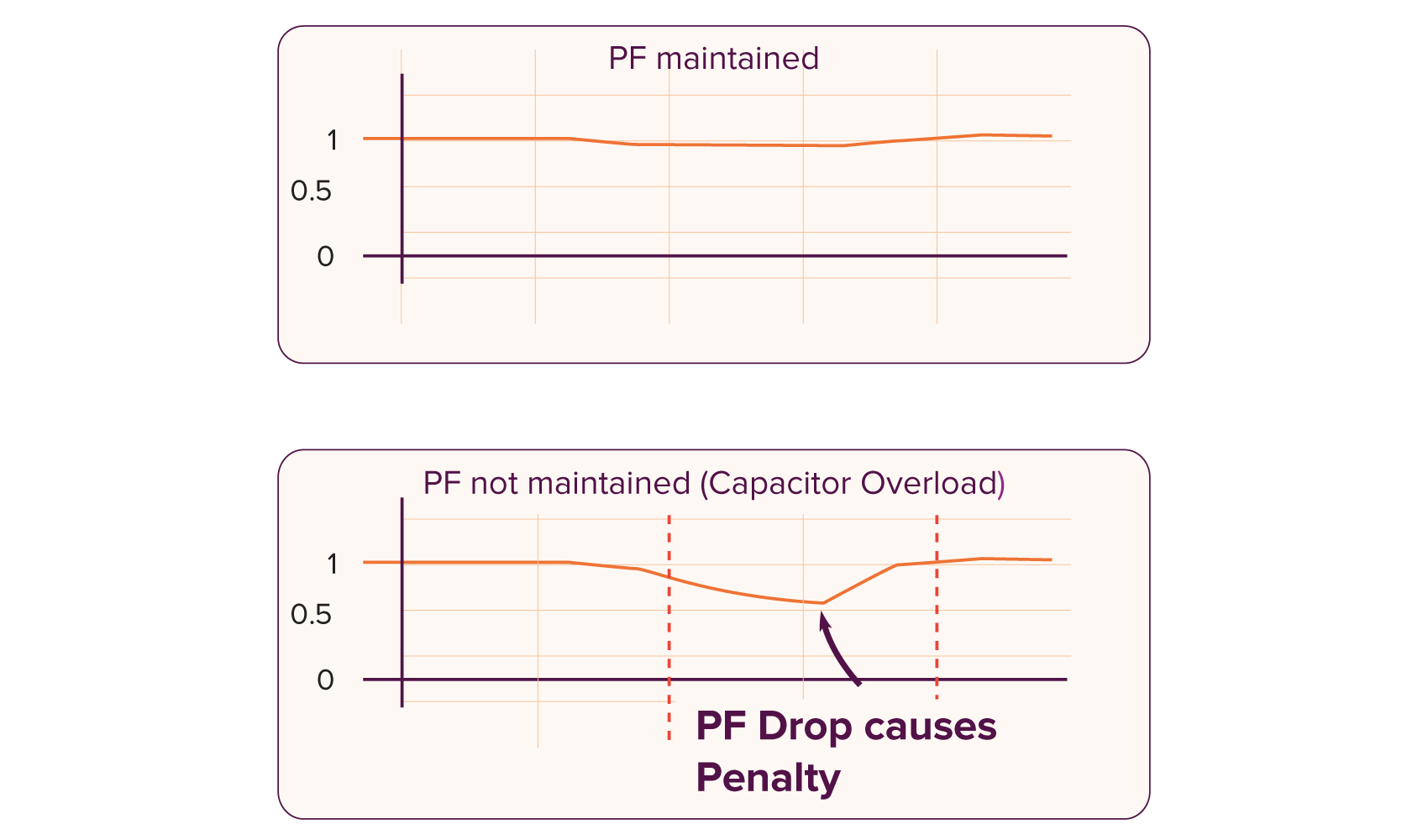#### Low Power Factor### What is Power Factor?

Power factor is the phase difference between the voltage and current. If the current leads the voltage it is called leading power factor. It occurs when there is a reactive power generation near the load. If the current lags the voltage, it is called lagging power factor. It occurs when there is a reactive power absorption by the load. Normally the leading power factor is prevented while lagging power factor is allowed to a certain extent.

Power factor (PF) is an expression of energy efficiency. PF is the ratio of working power to apparent power. Apparent power, also known as demand, is the measure of the amount of power used to run machinery and equipment during a certain period.PF expresses the ratio of true power used in a circuit to the apparent power delivered to the circuit.